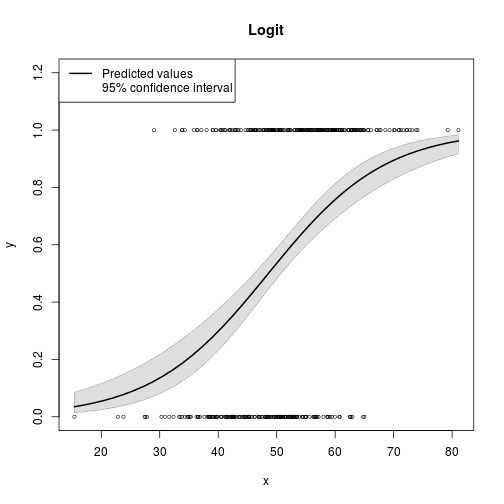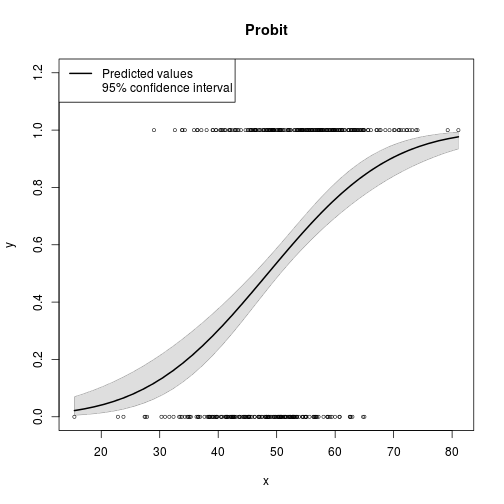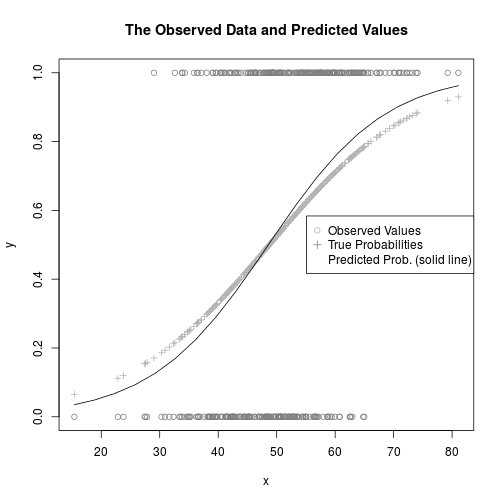## Paul Johnson
## 2014-05-02
## Understanding predicted values and creating confidence
## interval for logistic regressions via glm.

## Ever notice that predict for lm has argument "interval"
## but predict for glm does not?

## For a long time, I wondered about what to do about that.  How get
## confidence intervals on predicted values from glm regressions? The
## predict.glm method has no argument interval="conf" or such.  I've
## been checking into that. Apparently, there are many competing
## methods for creating confidence intervals on predicted values. Some
## programs simply ignore the controversy, asserting that one
## particular method ought to be used. Others provide a laundry list
## of methods. Others, avoid it.  I'll make a list, add to the
## end of this code. Will do later.

## Step one is to understand what predict.lm does, then see
## what we can do about predict.glm.

## make up some data suitable for logistic regression.

B0 = -3.9
B1 = 0.08

x <- sort(rnorm(400, m = 50, sd = 10))
eta <- B0 + B1 * x
pr <- 1/(1 + exp(-eta))
y <- rbinom(400, p = pr, size = 1)

dat <- data.frame(x = x, y = y, eta = eta)
rm(x, y, eta) ## clean up!
plot(pr ~ eta, data = dat,  main = "Underlying Nonlinear Model", type = "l", ylim = c(0,1))
points(y ~ eta, data = dat)m1 <- lm(y ~ x, data = dat)
plot(y ~ x, data = dat, main = "Observed Values With Linear Model")
abline(m1, col = "red")

library(rockchalk)

## Loading required package: MASS
##
## Attaching package: 'rockchalk'
##
## The following object is masked from 'package:MASS':
##
##     mvrnormnd <- newdata(m1, predVals = list(x = plotSeq(dat$x, 20))) nd[ , c("m1.fit", "m1.lwr", "m1.upr")] <- predict(m1, newdata = nd, type = "response", interval = "conf") m2a <- glm(y ~ x, data = dat, family = binomial(link = "logit")) plotCurves(m2a, plotx = "x", interval = "confidence", main = "Logit")  ## rockchalk:::predCI: model's predict method does not return an interval. We will improvize with a Wald type approximation to the confidence intervalm2b <- glm(y ~ x, data = dat, family = binomial(link = "probit")) plotCurves(m2b, plotx = "x", interval = "confidence", main = "Probit")  ## rockchalk:::predCI: model's predict method does not return an interval. We will improvize with a Wald type approximation to the confidence intervaloutreg(list("OLS" = m1, "Logit" = m2a, "Probit" = m2b), type = "html", showAIC = TRUE)  ##  "whew, there is a DF" ##  "whew, there is a DF" ##  "whew, there is a DF" ## ## We are launching a browser to view that html file, which we have temporarily ## saved in /tmp/RtmpDXr19W/file5a98fa67f95.html ## You can copy that temp file, or create ## one of your own with R's cat function. Like this: ## myreg <- ## outreg(modelList = list(OLS = m1, Logit = m2a, Probit = m2b), ## type = "html", showAIC = TRUE) ## ## cat(myreg, file = "reg.html") ## Then open reg.html in a word processor or web browser.  m2alink <- predict(m2a, newdata = nd, type = "link", se.fit = TRUE) m2alink <- as.data.frame(m2alink) colnames(m2alink) <- paste("m2alink.", colnames(m2alink), sep ="") nd <- cbind( nd, m2alink) plot(m2alink.fit ~ x, data = nd, type = "l", main = "The Linear Predictor") curve(B0 + B1 * x, from = min(nd$x), to = max(nd\$x), lty = 2, add = TRUE)
legend("bottomright", legend = c("True", "Estimated"),  lty = 1:2)m2aresp <- predict(m2a, newdata = nd, type = "response",  se.fit = TRUE)
m2aresp <- as.data.frame(m2aresp)
colnames(m2aresp) <- paste("m2aresp.", colnames(m2aresp), sep ="")
nd <- cbind(nd, m2aresp)

plot(y ~ x, data = dat, col = gray(.50), main = "The Observed Data and Predicted Values")
points(pr ~ x, data = dat, col = gray(.70), cex = 0.8, pch = 3)
lines(m2aresp.fit ~ x, data = nd)
legend("right", legend = c("Observed Values", "True Probabilities", "Predicted Prob. (solid line)"), col = c(gray(.70), gray(.50), 0), pch = c(1,3,0), bg = "white")##May help to look at that:
View(nd)

plot(m2aresp.fit ~ m2alink.fit, data = nd)